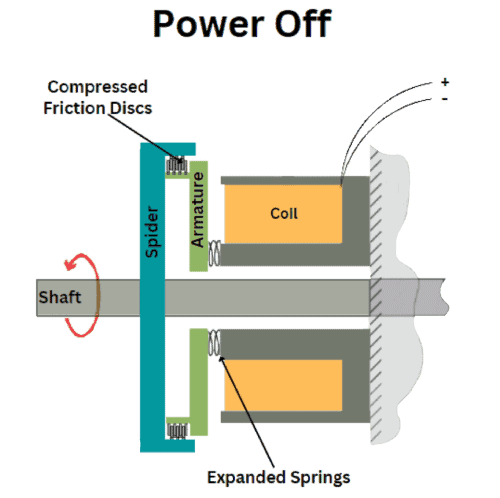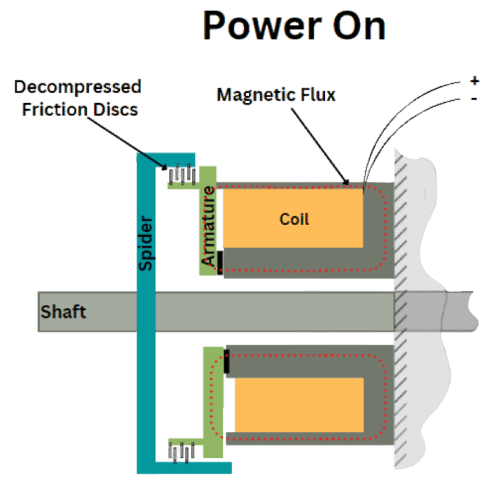# Multiple Disc Spring Applied Brake

## Multiple Disc Spring Applied Brake

• Wet Static Torque – 45 to 455 lb-ft
• Wet Starting Torque – 25 to 252 lb-ft
• Dry Static Torque – 50 to 506 lb-ft
• Dry Starting Torque – 38 to 378 lb-ft
• Three standard frame sizes
• Magnet Body Dia. – 5.2″ to 9.2″
• Overall Length – 3″ to 5.9″
• Bore sizes – 1.4″ to 3.5″

### Resources

Questions? (607) 732-2030
Performance / Mechanical Specifications MSB Series - Model Size
520 720 925
STP File Model
Model: 5203D
Model: 7203D
Model: 9253D
Model:
Model:
Model:
Model:
Model:
Model:
Torque Rating (Static-Wet) lb-ft
Model: 520
45
Model: 720
180
Model: 925
455
Model:
Model:
Model:
Model:
Model:
Model:
(Nm)
Model: 520
(61.1)
Model: 720
(244)
Model: 925
(611.9)
Model:
Model:
Model:
Model:
Model:
Model:
Torque Starting (Wet) lb-ft
Model: 520
25
Model: 720
100
Model: 925
252
Model:
Model:
Model:
Model:
Model:
Model:
(Nm)
Model: 520
(33.9)
Model: 720
(135.6)
Model: 925
(341.7)
Model:
Model:
Model:
Model:
Model:
Model:
Torque Rating (Static-Dry) lb-ft
Model: 520
50
Model: 720
200
Model: 925
506
Model:
Model:
Model:
Model:
Model:
Model:
(Nm)
Model: 520
(67.9)
Model: 720
(271.2)
Model: 925
(686.1)
Model:
Model:
Model:
Model:
Model:
Model:
Torque Starting (Dry) lb-ft
Model: 520
38
Model: 720
150
Model: 925
378
Model:
Model:
Model:
Model:
Model:
Model:
(Nm)
Model: 520
(51.50
Model: 720
(203.4)
Model: 925
(512.5)
Model:
Model:
Model:
Model:
Model:
Model:
Overall Length (ref) in
Model: 520
3.08
Model: 720
4.16
Model: 925
5.9
Model:
Model:
Model:
Model:
Model:
Model:
(mm)
Model: 520
(78.2)
Model: 720
(105.7)
Model: 925
(149.9)
Model:
Model:
Model:
Model:
Model:
Model:
Body Diameter in
Model: 520
5.19
Model: 720
7.16
Model: 925
9.25
Model:
Model:
Model:
Model:
Model:
Model:
(mm)
Model: 520
(131.8)
Model: 720
(181.9)
Model: 925
(234.9)
Model:
Model:
Model:
Model:
Model:
Model:
Magnet Body Co-Bore Diameter in
Model: 520
2.5
Model: 720
3.5
Model: 925
5.0
Model:
Model:
Model:
Model:
Model:
Model:
(mm)
Model: 520
(63.5)
Model: 720
(88.9)
Model: 925
(127)
Model:
Model:
Model:
Model:
Model:
Model:

## MSB Operation

This spring applied brake is designed specifically for applications requiring braking when power fails or when the brake will be engaged for long periods of time. Spring applied clutches also available. Contact SEPAC Engineering for details. Many options are available.
When current is applied to the brake, the armature is attracted toward the magnet body releasing the discs, allowing them to rotate. When current is cut to the coil, springs push the armature into engagement with the discs stopping the load.